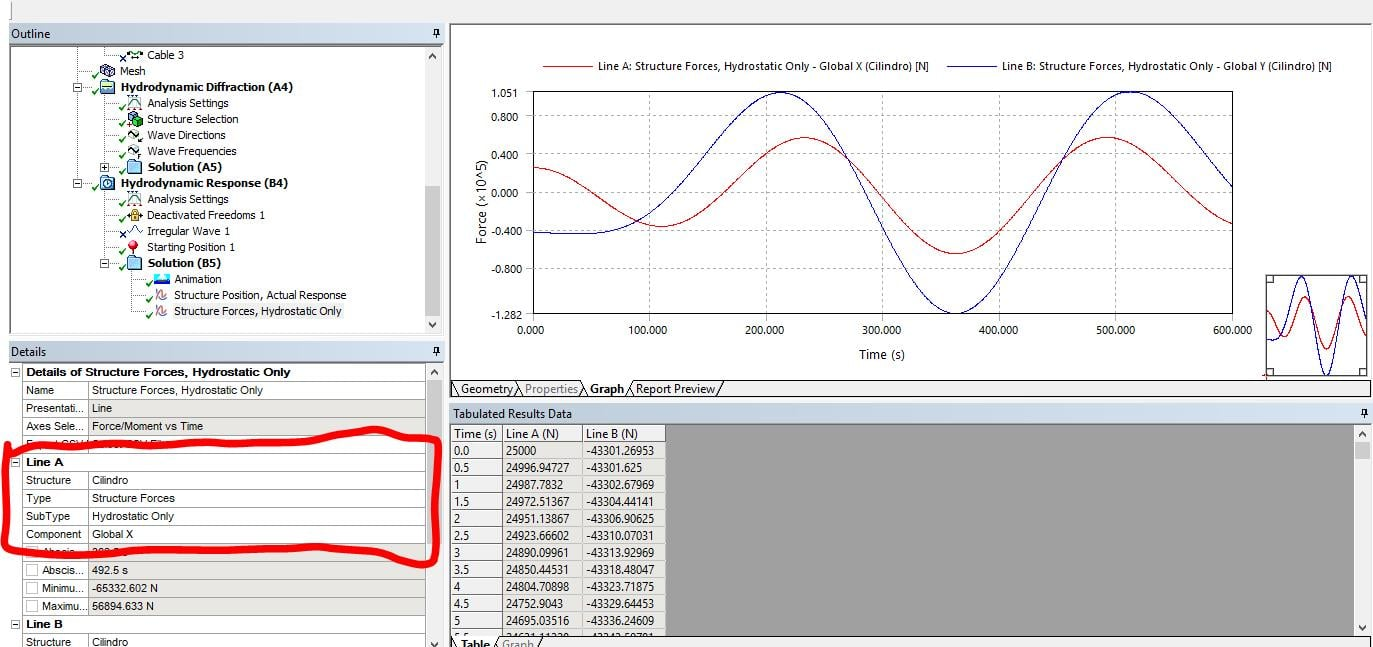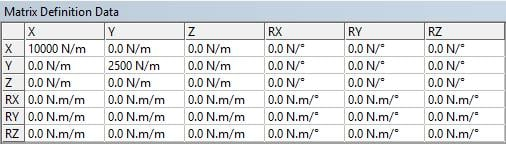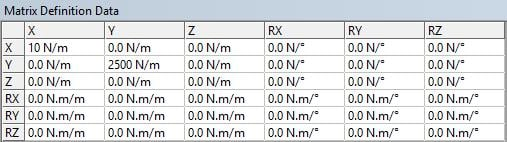## General Mechanical

•FCNQP
Subscriber

Good afternoon,

I am currently modelling a floating structure with ANSYS Aqwa module and I believe there is an incoherence in this when considering the hydrostatic stiffness of the structure. I've run a test in a cylinder where the only restoring surge and sway forces are given by an added stiffness of 10000 and 2500 N/m, respectively and all other degrees of freedom are blocked. Now, I have inserted an initial displacement in the sway direction of 20 [m] and the forces are correct (20 x 2500 = 50000 [N]). But when I add an initial condition RZ=30º things don't seem to work. As I was trying to understand if the stiffness matrix rotates with the platform I believe two different things can happen:

1 - If the matrix is defined in the global axis

In this case, the results should be the same as in the situation with RZ=0º

2 - If the matrix is defined in the local axis

In this case, the forces should be computed with the respective displacement in the local axis and corresponding stiffness.

However neither seems to happen.

•FCNQP
Subscriber

The magnitude of the forces is computed as if the matrix is written in the global axis and then transformed to the local axis, so I get:

FX=2500 x 20 x sin(30º )=25000

FY=-2500 x 20 x cos(30º )=-43301.3

I don't believe this makes any sense because the forces written in this way are not in the global system as it is said (see figure below).With this in mind I think the forces should be one of the following:

1 - If the matrix is defined in the global axis

FY=2500 x 20=50000

FX=0

2 - If the matrix is defined in the local axis (forces in global axis)

FX=-10000 x 20 x sin(30º ) x cos(30º )+2500 x 20 x sin(30º ) x cos(30º )=64951.9

FY=-10000 x 20 x sin(30º ) x sin(30º )-2500 x 20 x cos(30º ) x cos(30º )=-87500

Am I misunderstanding something or is there really an incoherence in the axis used by ANSYS Aqwa?

•Sandeep Medikonda
Ansys Employee
•FCNQP
Subscriber

I thought so, but the results do not match the expected values. Please, consider the image I attached and that is repeated below:As you can see, in the first time step, the forces are not correct (I made an error in the previous post where I did not consider both directions of the stifness matrix but the conclusions remain the same. I have edited the post to include all the forces and the results are now the same as yours calculations. The results obtained in a simulation are not, as you can see in the picture above.)

Another example of this problem can be found when calculating the forces with different stiffness matrix:

1 - The matrix presented before - Matrix 1: k11=10000; k22=2500;2 - Matrix 2 before: k11=10; k22=2500;In both cases the forces are exactly the same at the first time step. I have also attached the file so you can check with you wish these results.

•pjschofi
Ansys Employee

It has been found that Aqwa is incorrectly utilizing the defined additional hydrostatic stiffness. A defect has been raised and development will investigate.

•claudiorc
Subscriber

Hello,

I am having a problem related to the above. In my case, I am modelling a floating body (a wave energy converter) that oscillates only along an inclined axis (relative to the horizontal plane). I have managed to represent this set-up in Aqwa by defining fenders that allow motions only along this inclined direction. The problem is that Aqwa only provides the motions and forces for the classical degrees of freedom (GLOBAL X, GLOBAL Y, GLOBAL, Z, GLOBAL RX, and so on). Since in the hydrodynamic diffraction computation of Aqwa, the hydrodynamic (and hydrostatic) forces are computed along the global degrees of freedom, I would like to know, if and how Aqwa computes (or transforms) the forces along the inclined axis for the time-domain (hydrodynamic response) computations, or if it just computes the forces and motions as if the floater were free to oscillate independently in surge and heave.

•claudiorc
Subscriber

It is not just a matter of composing GLOBAL X and GLOBAL Z forces or motions, because in my case heave and pitch are not independent of each other.  Based on experimental results and numerical simulations with another hydrodynamic software (Wamit), we know that inclination affects the resultant hydrodynamic (and hydrostatic) forces along the inclined direction, so that the period of motion is affected.

Similar, to the problem described above for the additional stiffness matrix when a rotation is applied, we would like to know if the same happens with the rotation of the hydrodynamic coefficients matrixes and the hydrostatic restoring matrix. How to obtain/define these forces/motions in a generic local axis?